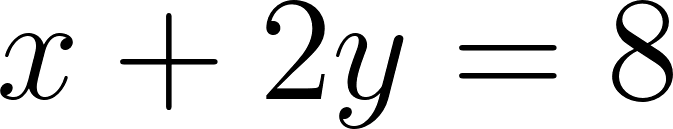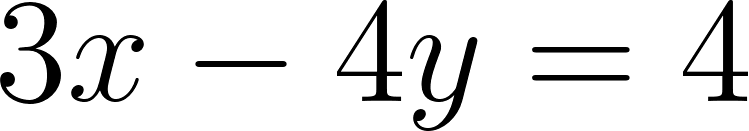×
Get Full Access to Elementary Linear Algebra With Applications - 9 Edition - Chapter 1.1 - Problem 1
Get Full Access to Elementary Linear Algebra With Applications - 9 Edition - Chapter 1.1 - Problem 1

×

# III EJerdse.\ Ilhrol/gh 14 . . \"O/re each gil'ell lillear ~)'~'Ielll by fhe It1nl/Od ofISBN: 9780132296540 301

## Solution for problem 1 Chapter 1.1

Elementary Linear Algebra with Applications | 9th Edition

• Textbook Solutions
• 2901 Step-by-step solutions solved by professors and subject experts
• Get 24/7 help from StudySoup virtual teaching assistantsElementary Linear Algebra with Applications | 9th Edition

4 5 1 315 Reviews
11
4
Problem 1

Solve each given linear system by the method of elimination.

x + 2y - 8

3x - 4y = 4

Step-by-Step Solution:

Problem 1

Solve each given linear system by the method of elimination.

x + 2y - 8

3x - 4y = 4

Step-by-step solution

Step 1 of  2

Let us consider ,.....(1)....(2)

Step 2 of 2

##### ISBN: 9780132296540

The answer to “?Solve each given linear system by the method of elimination.x + 2y - 83x - 4y = 4” is broken down into a number of easy to follow steps, and 18 words. This full solution covers the following key subjects: . This expansive textbook survival guide covers 57 chapters, and 1519 solutions. The full step-by-step solution to problem: 1 from chapter: 1.1 was answered by , our top Math solution expert on 01/30/18, 04:18PM. Elementary Linear Algebra with Applications was written by and is associated to the ISBN: 9780132296540. Since the solution to 1 from 1.1 chapter was answered, more than 298 students have viewed the full step-by-step answer. This textbook survival guide was created for the textbook: Elementary Linear Algebra with Applications, edition: 9.

## Discover and learn what students are asking

Calculus: Early Transcendental Functions : Product and Quotient Rules and Higher-Order Derivatives
?Using the Product Rule In Exercises 1–6, use the Product Rule to find the derivative of the function. \(g(x)=\left(x^{2}+3\right)\left(x^{2}-4 x\

Statistics: Informed Decisions Using Data : Scatter Diagrams and Correlation
?A _______ _______ is a graph that shows the relation between two quantitative variables.

Unlock Textbook Solution